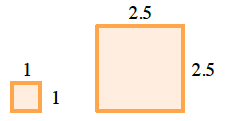### Home > CC1 > Chapter 5 > Lesson 5.1.4 > Problem5-35

5-35.

Draw a rectangle. Label the lengths of the sides. Enlarge it so that the ratio of sides of your new rectangle to the original one is $\frac { 5 } { 2 }$. What are the new dimensions? Homework Help ✎

Fitzwilliam was asked to draw two squares with the ratio of sides 2:5.
As you can see, each side of the original square is 1 unit, and each side of the new square is 2.5 units.
Can you find a way that Fitzwilliam may have enlarged his squares?$\text{Fitzwilliam multiplied each side by 2.5, or }\frac{5}{2},\text{ in order to enlarge his square.}$

Do you think this will work for your rectangles?
Make sure your drawings are neat!